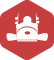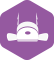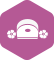### 如何将Matlab三维点云图中两个独立分开的点云集团分离开来

C/C++ > 其它技术问题 [问题点数：100分]2012年 总版技术专家分年内排行榜第七2012年 总版技术专家分年内排行榜第七2012年 总版技术专家分年内排行榜第七cchcjl

MATLAB点云处理：读取、展示、最近邻、ICP算法求取转移矩阵、旋转

MATLAB中关于点云的几函数的简单应用。作者使用的是MATLAB R2015b，这几函数应该是在Computer Vison包里。 全文都是作者自己结合MATLAB文档的理解，欢迎指教。 1. pcread: 输入文件名，返回pointCloud类（用于...

A = load('cloud3d.dat'); %读入数据 %x,y,z轴坐标 x=A(:,1); y=A(:,2); z=A(:,3);...[X,Y,Z]=griddata(x,y,z,linspace(min(x),max(x))',linspace(min(y),max(y)),'v4'); %构造坐标 pcolor(X,Y,

MATLAB点云处理基本函数

*三维点图像转化为三维模型，Title_MapX等为包含三维信息的图像，不是信息 xyz_to_object_model_3d(Title_MapX, Title_MapY, Title_MapZ, Model3D) *报错xyz_to_object_model_3d(CoordX, CoordY, CoordZ, Model) ...

matlab将深度图转为点云

matlab将深度图转为点云显示的代码： intrinsic=[fx,0,cx  0,fy,cy  0,0,1]; fdx=intrinsic(1,1); fdy=intrinsic(2,2); u0=intrinsic(1,3); v0=intrinsic(2,3); [h,w] = size(imdepth); u=repmat...

matlab读入三维点云数据斯坦福兔子

openGL显示3d 点云图像

1.深度图像也叫距离影像，是指从图像采集器到场景的距离（深度）值作为像素值的图像。获取方法有：激光雷达深度成像法、计算机立体视觉成像、坐标测量机法、莫尔条纹法、结构光法。 2.点云：当一束激光照射...

PCL编程->三维彩色点云显示

【python】处理点云数据并三维显示

import numpy as np import matplotlib.pyplot as plt ...#打开点云数据文件 f=open('RawXYZ.xyz','r') point=f.read() f.close() #数据预处理 l1=point.replace('\n',',') #数据以“，”进行分割...

【仓库物资识别】四、将三维点云转换成二图像（修正后）

RealSense D435 点云的获取与处理

SICK LMS511 激光雷达获取的数据怎么建立三维点云图

【雷达】如何将三维点云映射到二平面

matlab点云生成，可以参考我的github ...使用matlab mex命令编译成功后，可以在matlab下调用generatePointsCloud函数，该函数接受5参数，分别是 1. faceIdx，该...

3D点云图

3D点云图  3D点云图用来表示3D扫描的原始数据点群，显示原始数据的初貌。  点云数据量一般比较大，... 本图主要有三个空间旋转角和扫描的原始数据组编号以及数据的型式、大小、颜色和表示的比例参数。  ...

Kinect深度图和彩图校准生成点云代码技术总结

RGB图和Depth图生成点云图原理与代码实现（realsense D435 ）

1、首先，看看四坐标系之间的转换关系，就明白了 ： dx dy 分别表示u轴和v轴方向上每像素的物理尺寸（图说法不正确） 2、代码如下，可直接运行： #include <iostream> #include <...

matlab（对点云的简单处理）